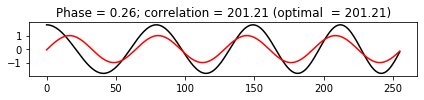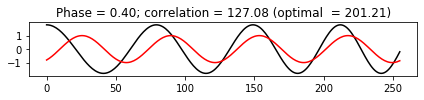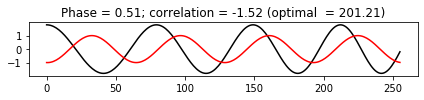Computing a discrete Fourier transform results in complex-valued Fourier coefficients. Each such coefficient can be represented by a magnitude and a phase component. Follow Section 2.3.2 of [Müller, FMP, Springer 2015], we discuss in this notebook the role of the phase component.

## Polar Representation of Fourier Coefficients¶

Let $x=(x(0), x(1), ..., x(N-1))$ be a signal with samples $x(n)\in\mathbb{R}$ for $n\in[0:N-1]$. The complex Fourier coefficient $c_k:=X(k)\in\mathbb{C}$ for $k\in[0:N-1]$, as computed by the discrete Fourier transform (DFT), is given by

$$c_k :=X(k) = \sum_{n=0}^{N-1} x(n) \exp(-2 \pi i k n / N).$$

Let $c_k = a_k + i b_k$ with real part $a_k\in\mathbb{R}$ and imaginary part $b_k\in\mathbb{R}$. Recall from the FMP notebook on complex numbers that the absolute value is defined by

$$|c_k| := \sqrt{a_k^2 + b_k^2}$$

and the angle (given in radians) by

$$\gamma_k := \mathrm{angle}(c_k) := \mathrm{atan2}(b_k, a_k) \in [0,2\pi).$$

Using the exponential function, this yields the polar coordinate representation

$$c_k = |c_k| \cdot \mathrm{exp}(i \gamma_k).$$

## Optimality Property¶

Let $\mathbf{cos}_{k,\varphi}:[0:N-1]\to\mathbb{R}$ be a sampled sinusoid of with frequency parameter $k$ and phase $\varphi\in[0,1)$, defined by

$$\mathbf{cos}_{k,\varphi}(n) = \sqrt{2}\mathrm{cos}\big( 2\pi (kn/N - \varphi) \big)$$

for $n\in[0,N-1]$. Intuitively speaking, when computing the Fourier transform for some discrete signal $x$ of length $N$ and for some frequency parameter $k$, one computes an inner product (a kind of correlation) of the signal $x$ and the sinusoid $\mathbf{cos}_{k,\varphi_k}$. The phase $\varphi_k$ has the remarkable property that it maximizes the correlation between $x$ and all possible sinusoids $\mathbf{cos}_{k,\varphi}$ with $\varphi\in[0,1)$. In other words:

$$\varphi_k = \mathrm{argmax}_{\varphi\in[0,1)} \langle x | \mathbf{cos}_{k,\varphi} \rangle.$$

The complex Fourier coefficient $X(k)$ encodes this optimal phase, which is basically given by the angle of the complex number. More precisely, let $\gamma_k$ be the angle of $X(k)$, then one can show that the optimal phase $\varphi_k$ is given by

$$\varphi_k := - \frac{\gamma_k}{2 \pi}.$$

In the following code cell, we demonstrate this optimality property.

In :
import os
import sys

import numpy as np
from matplotlib import pyplot as plt

sys.path.append('..')
import libfmp.b
import libfmp.c2
import libfmp.c6

%matplotlib inline

# Generate a chirp-like test signal (details not important)
N = 256
t_index = np.arange(N)
x = 1.8 * np.cos(2 * np.pi * (3 * (t_index * (1 + t_index / (4 * N))) / N))

k = 4
exponential = np.exp(-2 * np.pi * 1j * k * t_index / N)
X_k = np.sum(x * exponential)
phase_k = - np.angle(X_k) / (2 * np.pi)

def compute_plot_correlation(x, N, k, phase):
sinusoid = np.cos(2 * np.pi * (k * t_index / N - phase))
d_k = np.sum(x * sinusoid)
plt.figure(figsize=(6,1.5))
plt.plot(t_index, x, 'k')
plt.plot(sinusoid, 'r')
plt.title('Phase = %0.2f; correlation = %0.2f (optimal  = %0.2f)' % (phase, d_k, np.abs(X_k)))
plt.tight_layout()
plt.show()

print('Sinusoid with phase from Fourier coefficient resulting in an optimal correlation.')
compute_plot_correlation(x, N, k, phase=phase_k)

print('Sinusoid with an arbitrary phase resulting in a medium correlation.')
compute_plot_correlation(x, N, k, phase=0.4)

print('Sinusoid with a phase that yields a correlation close to zero.')
compute_plot_correlation(x, N, k, phase=0.51)

Sinusoid with phase from Fourier coefficient resulting in an optimal correlation.Sinusoid with an arbitrary phase resulting in a medium correlation.Sinusoid with a phase that yields a correlation close to zero.Acknowledgment: This notebook was created by Meinard Müller.